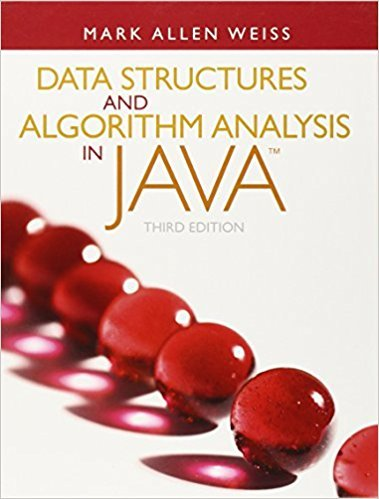×
Get Full Access to Data Structures And Algorithm Analysis In Java - 3 Edition - Chapter 6 - Problem 6.33
Get Full Access to Data Structures And Algorithm Analysis In Java - 3 Edition - Chapter 6 - Problem 6.33

×

# a. Show that N inserts into an initially empty binomial queue takes O(N) time intheISBN: 9780132576277 316

## Solution for problem 6.33 Chapter 6

Data Structures and Algorithm Analysis in Java | 3rd Edition

• Textbook Solutions
• 2901 Step-by-step solutions solved by professors and subject experts
• Get 24/7 help from StudySoup virtual teaching assistantsData Structures and Algorithm Analysis in Java | 3rd Edition

4 5 1 363 Reviews
19
3
Problem 6.33

a. Show that N inserts into an initially empty binomial queue takes O(N) time inthe worst case.b. Give an algorithm to build a binomial queue of N elements, using at most N 1comparisons between elements. c. Propose an algorithm to insert M nodes into a binomial queue of N elements inO(M + logN) worst-case time. Prove your bound.

Step-by-Step Solution:
Step 1 of 3

The Human Body: An Orientation ● Anatomy: ​ structure of the parts that make up the body ● Physiology:​ function of those parts and how they work together ● Parts of the Heart: ○ Pulmonary Trunk ○ Superior Vena Cava ○ Ascending Aorta ○ Right Atrium ○ Left Ventricle ○ Right Ventricle ○ Inferior Vena Cava ○ Descending Aorta ● Survival Needs: ○ Nutrients: ​chemicals for energy and cell building (carbohydrates, proteins, lipids, vitamins, and minerals) ○ Oxygen: ​ required for every cell in the body ● Homeostasis​ : maintenance of a balanced environment ○ Dynamic state of equilibrium ○ Necessary to sustain life ○ Built in responsive systems ○ Homeostatic imbalance or disturbance leads to disease ● Feedback Mechanisms ■ Negative Feedback: reduces stimulus intensity ■ Positive Feedback: increases stimulus intensity (ie. blood clotting, child­ birth) Language of Anatomy (pg. 15) ● Used for position, direction, regions, structures ○ Superior ○ Inferior ○ Ventral ○ Dorsal ○

Step 2 of 3

Step 3 of 3

##### ISBN: 9780132576277

Data Structures and Algorithm Analysis in Java was written by and is associated to the ISBN: 9780132576277. The answer to “a. Show that N inserts into an initially empty binomial queue takes O(N) time inthe worst case.b. Give an algorithm to build a binomial queue of N elements, using at most N 1comparisons between elements. c. Propose an algorithm to insert M nodes into a binomial queue of N elements inO(M + logN) worst-case time. Prove your bound.” is broken down into a number of easy to follow steps, and 58 words. This textbook survival guide was created for the textbook: Data Structures and Algorithm Analysis in Java, edition: 3. The full step-by-step solution to problem: 6.33 from chapter: 6 was answered by , our top Science solution expert on 03/02/18, 06:05PM. This full solution covers the following key subjects: . This expansive textbook survival guide covers 12 chapters, and 457 solutions. Since the solution to 6.33 from 6 chapter was answered, more than 236 students have viewed the full step-by-step answer.

## Discover and learn what students are asking

Calculus: Early Transcendental Functions : Riemann Sums and Definite Integrals
?In Exercises 3–8, evaluate the definite integral by the limit definition. $$\int_{-2}^{3} x d x$$

Statistics: Informed Decisions Using Data : Comparing Three or More Means (One-Way Analysis of Variance)
?Soybean Yield The data in the table represent the number of pods on a random sample of soybean plants for various plot types. An agricultural research

Statistics: Informed Decisions Using Data : Testing the Significance of the Least-Squares Regression Model
?In Problems 5–10, use the results of Problems 7–12, respectively, from Section 4.2 to answer the following questions: (a) What are the estima

Unlock Textbook Solution